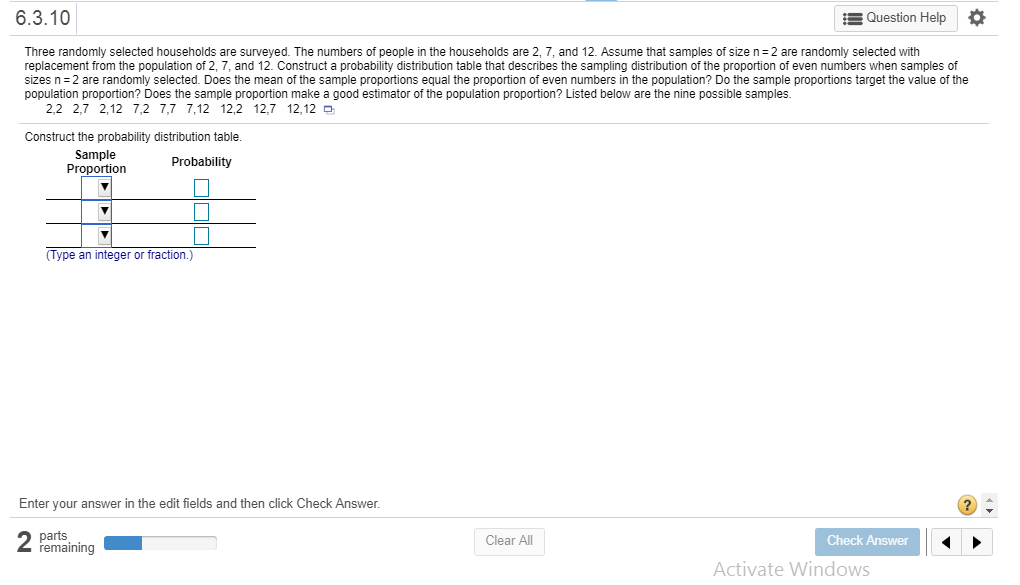# 6.3.10Question HelpThree randomly selected households are surveyed. The numbers of people in the households are 2, 7, and 12. Assume that samples of size n 2 are randomly selected withreplacement from the population of 2, 7, and 12. Construct a probability distribution table that describes the sampling distribution of the proportion of even numbers when samples ofsizes n 2 are randomly selected. Does the mean of the sample proportions equal the proportion of even numbers in the population? Do the sample proportions target the value of thepopulation proportion? Does the sample proportion make a good estimator of the population proportion? Listed below are the nine possible samples.2,2 2,7 2,12 7,2 7,7 7,12 12,2 12,7 12,12Construct the probability distribution table.SampleProportionProbabilityType an integer or fraction.)Enter your answer in the edit fields and then click Check Answer.partsremainingClear AllCheck AnswerActivate Windows

Questionhelp_outlineImage Transcriptionclose6.3.10 Question Help Three randomly selected households are surveyed. The numbers of people in the households are 2, 7, and 12. Assume that samples of size n 2 are randomly selected with replacement from the population of 2, 7, and 12. Construct a probability distribution table that describes the sampling distribution of the proportion of even numbers when samples of sizes n 2 are randomly selected. Does the mean of the sample proportions equal the proportion of even numbers in the population? Do the sample proportions target the value of the population proportion? Does the sample proportion make a good estimator of the population proportion? Listed below are the nine possible samples. 2,2 2,7 2,12 7,2 7,7 7,12 12,2 12,7 12,12 Construct the probability distribution table. Sample Proportion Probability Type an integer or fraction.) Enter your answer in the edit fields and then click Check Answer. parts remaining Clear All Check Answer Activate Windows fullscreen
check_circleExpert Solution
Step 1

Sample proportions:

The sample proportion of even numbers = (number of even numbers in the sample of two households)/2.

For example, for observations (2, 2), there are 2 even numbers in the sample of two households. So, corresponding proportion of even numbers is: 2/2 = 1.

Again, for the observations (2, 7), there is 1 odd number and 1 even number. So, proportion of even numbers is: 1/2.

The following table lists the possible samples with replacement, from the three given observations, along with the sample proportion of even numbers for each sample:

Step 2

Probability distribution:

Observe that, there are a total of 9 possible samples. In the 9 samples, the proportion 0 occurs 1 time. So, the probability of proportion 0 is 1/9.

The proportion 1/2 occurs 4 times in 9 samples, so that the probability of the proportion 1/2 is 4/9.

Now, each of the proportion 1 occurs 4 times in 9 samples, so that the probability of the proportion 1 is 4/9.

The total probability is: 1/9 + 4/9 + 4/9 = 9/9 = 1.

Using these probabilities, the probability distribution of the sample proportions is shown as follows:

Step 3

Explanation:

The mean of the sample proportions is: p = (0 × 1/9) + (1/2 × 4/9) + (1 × 4/9) = 6/9 = 2/3.

In the population, there are 2 even numbers and 1 odd number, out of the three numbers. So, the population proportion of even numbers is 2/3.

Evidently, the mean of the sample proportions is equal to the population proportion of even numbers.

Thus, the sample proportion targets to find the population proportion.

Now, the mean of the samp...

### Want to see the full answer?

See Solution

#### Want to see this answer and more?

Solutions are written by subject experts who are available 24/7. Questions are typically answered within 1 hour*

See Solution
*Response times may vary by subject and question
Tagged in

### Other